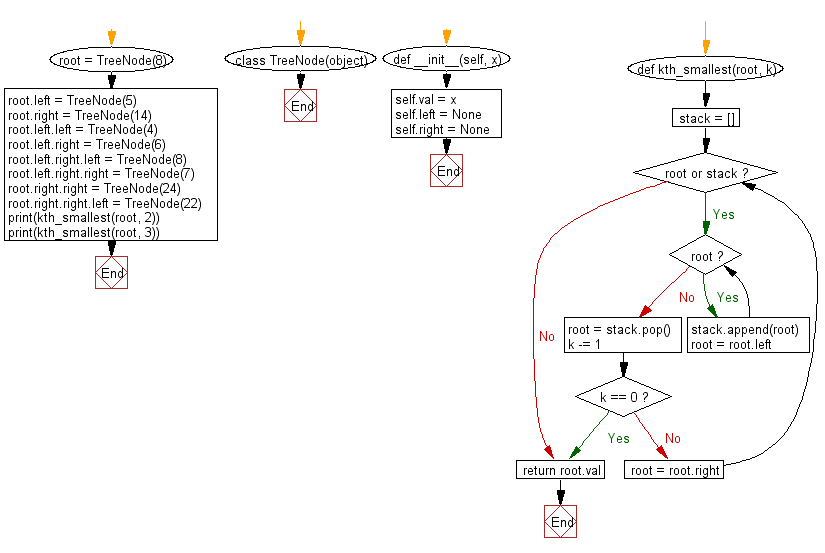﻿ Python Binary Search Tree: Find the kth smallest element in a given a binary search tree(BST) - w3resource# Python Binary Search Tree: Find the kth smallest element in a given a binary search tree (BST)

## Python Binary Search Tree: Exercise-6 with Solution

Write a Python program to find the kth smallest element in a given a binary search tree.

Sample Solution:

Python Code:

``````class TreeNode(object):
def __init__(self, x):
self.val = x
self.left = None
self.right = None

def kth_smallest(root, k):
stack = []
while root or stack:
while root:
stack.append(root)
root = root.left
root = stack.pop()
k -= 1
if k == 0:
break
root = root.right
return root.val

root = TreeNode(8)
root.left = TreeNode(5)
root.right = TreeNode(14)
root.left.left = TreeNode(4)
root.left.right = TreeNode(6)
root.left.right.left = TreeNode(8)
root.left.right.right = TreeNode(7)
root.right.right = TreeNode(24)
root.right.right.left = TreeNode(22)

print(kth_smallest(root, 2))
print(kth_smallest(root, 3))
```
```

Sample Output:

```5
8
```

Flowchart:Python Code Editor:

What is the difficulty level of this exercise?

Test your Python skills with w3resource's quiz

﻿

## Python: Tips of the Day

Python: The Zip() Function

```>>> students = ('John', 'Mary', 'Mike')
>>> ages = (15, 17, 16)
>>> scores = (90, 88, 82, 17, 14)
>>> for student, age, score in zip(students, ages, scores):
...     print(f'{student}, age: {age}, score: {score}')
...
John, age: 15, score: 90
Mary, age: 17, score: 88
Mike, age: 16, score: 82
>>> zipped = zip(students, ages, scores)
>>> a, b, c = zip(*zipped)
>>> print(b)
(15, 17, 16)
```